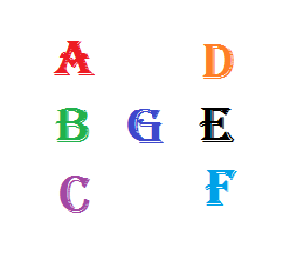Equal Products

Logic Level 2In the figure above, each letter represents a distinct single digit among $0, 1, 2, \ldots, 9.$ They are positioned in such a way that $\large A\times B\times C=B\times G\times E=D\times E\times F.$

What digit does the letter $G$ represent?

×